# Shape Detector

This is a simple tool that predicts drawing drawn on the canvas. It uses CNN to recognize the drawings. The CNN in trained on Quick, Draw! dataset. Trained model’s weights are used to make inference on the browser using `Tensorflow.js`.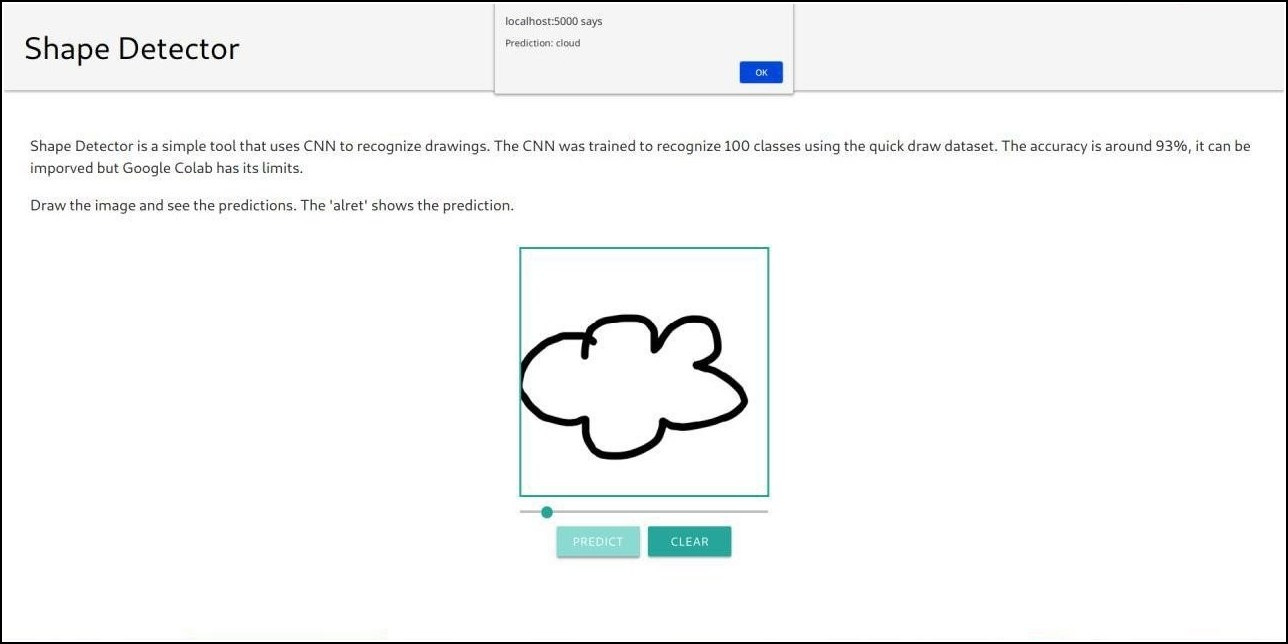You can find the demo here and source code here.

## Model

The Model will be using `Keras` with `Tensorflow` backend. The Model was built with `Sequential Api` of Keras followed which the Model and weights are converted into `Tensorflow.js`. Here are the steps that we would follow as to build our model :-

1. We will be working on only `100 classes` due to limited resources. Data on `Google cloud` includes `345 classes` So to fetch only 100 classes we will download a text file named `mini_classes.txt`. To do so linux user can directly download it via `wget` where others can directly go to the link and save the file with `Ctrl+s`.

`wget -O mini_classes.txt https://raw.githubusercontent.com/rohit3463/shape-detector/master/public/mini_classes.txt`

The mini_classes.txt file and the python file should be in the same directory.

2. Importing the essential packages.

``````import os
import glob
import numpy as np
from tensorflow.keras import layers
import tensorflow as tf
from tensorflow import keras
import urllib.request
import tensorflowjs as tfjs
import shutil``````

3. Downloading the Data from `GCP`.

``````f = open("mini_classes.txt","r")
f.close()

classes = [c.replace('\n','').replace(' ','_') for c in classes]
os.makedirs('data')

for c in classes:
cls_url = c.replace('_', '%20')
path = base+cls_url+'.npy'
print(path)
urllib.request.urlretrieve(path, 'data/'+c+'.npy')

def load_data(root, vfold_ratio = 0.2, max_items_per_class = 5000):
#all_files will contain list of all the files in data directory.
all_files = glob.glob(os.path.join(root,'*.npy'))

#initialize the variables with empty arrays and list respectively.
x = np.empty([0, 784])
y = np.empty()
classes = []

#enumerate all the files then load each file in a numpy array following which it is concatenated to the x and y arrays.
for idx, files in enumerate(all_files):
data = data[0:max_items_per_class,:]
labels = np.full(data.shape,idx)
x = np.concatenate((x, data), axis = 0)
y = np.append(y,labels)

class_name, ext = os.path.splitext(os.path.basename(files))
classes.append(class_name)

data = None
labels = None

#shuffle the data.
permutation = np.random.permutation(y.shape)

x = x[permutation,:]
y = y[permutation]

#prepare the testing and training set.
vfold_size = int(x.shape/100*(vfold_ratio*100))

x_test = x[:vfold_size,:]
y_test = y[:vfold_size]

x_train = x[vfold_size:x.shape,:]
y_train = y[vfold_size:y.shape]

#return the test and train sets along with their classes
return x_test, y_test, x_train, y_train, classes

x_test, y_test, x_train, y_train, class_names = load_data('data')
num_classes = len(class_names)
image_size = 28``````

4. Preprocessing is a very important step. It is the way we prepare the ingredients to be added to our dish by washing, cutting veggies. Our model takes training data of shape `[N, 28, 28, 1]`.

``````#reshaping the data into [N, 28, 28, 1]
x_train = x_train.reshape(x_train.shape, image_size, image_size, 1).astype('float32')
x_test = x_test.reshape(x_test.shape, image_size, image_size, 1).astype('float32')

#normalizing the data
x_train /= 255.0
x_test /= 255.0

#changing targets to categorical among 100 classes
y_train = keras.utils.to_categorical(y_train, num_classes)
y_test = keras.utils.to_categorical(y_test, num_classes)``````

5. Finally, the wait is over. Now, we will build our sequential model using simple `CNN` along with some `Maxpool layers` to extract the best features.In the end, we will be using `fully connected layers` to shape the output into 100 classes. Here comes the model:-

``````model = keras.Sequential()

print(model.summary())
model.fit(x = x_train, y = y_train, validation_split=0.1, batch_size = 256, verbose=2, epochs=5)``````

6. Evaluating the model describes how well our model performes. This is where test test will come in use.

``````score = model.evaluate(x_test, y_test, verbose=0)
print(score)``````

7. Saving the model by importing into `tensorflow.js`.

``````#converting the model into tensorflow.js and saving into a folder named 'SavedModel'.
tfjs.converters.save_keras_model(model, './SavedModel')

#writting all the class_names into a text file.
with open('class_names.txt', 'w') as file_handler:
for item in class_names:
file_handler.write("{}\n".format(item))

#copying the class_names.txt file into our Model and then zipping it.
shutil.copy('class_names.txt', './SavedModel')
shutil.make_archive('Model', 'zip', 'SavedModel')``````

That’s it for the model. The model was actually trained on `Google Colab` where it took around `25-30 mins` to train the model. Now, proceeding to the frontend.

## Frontend

The frontend is written in `Vue.js` using single-file components. The weights obtained from the training part are used for inference via `Tensorflow.js`. The boilerplate for vue is generated via `vue-cli` using only the `babel` plugin. `Fabric.js` is used for canvas drawing and management. It is a simple and powerful HTML `canvas` library. I am assuming that you have a working knowledge of Vue.js.

```Root
└───App
│   Title
│   Description
└───Canvas
RangeSlider
CustomButton
CustomButton```

We have the above components with hierarchy.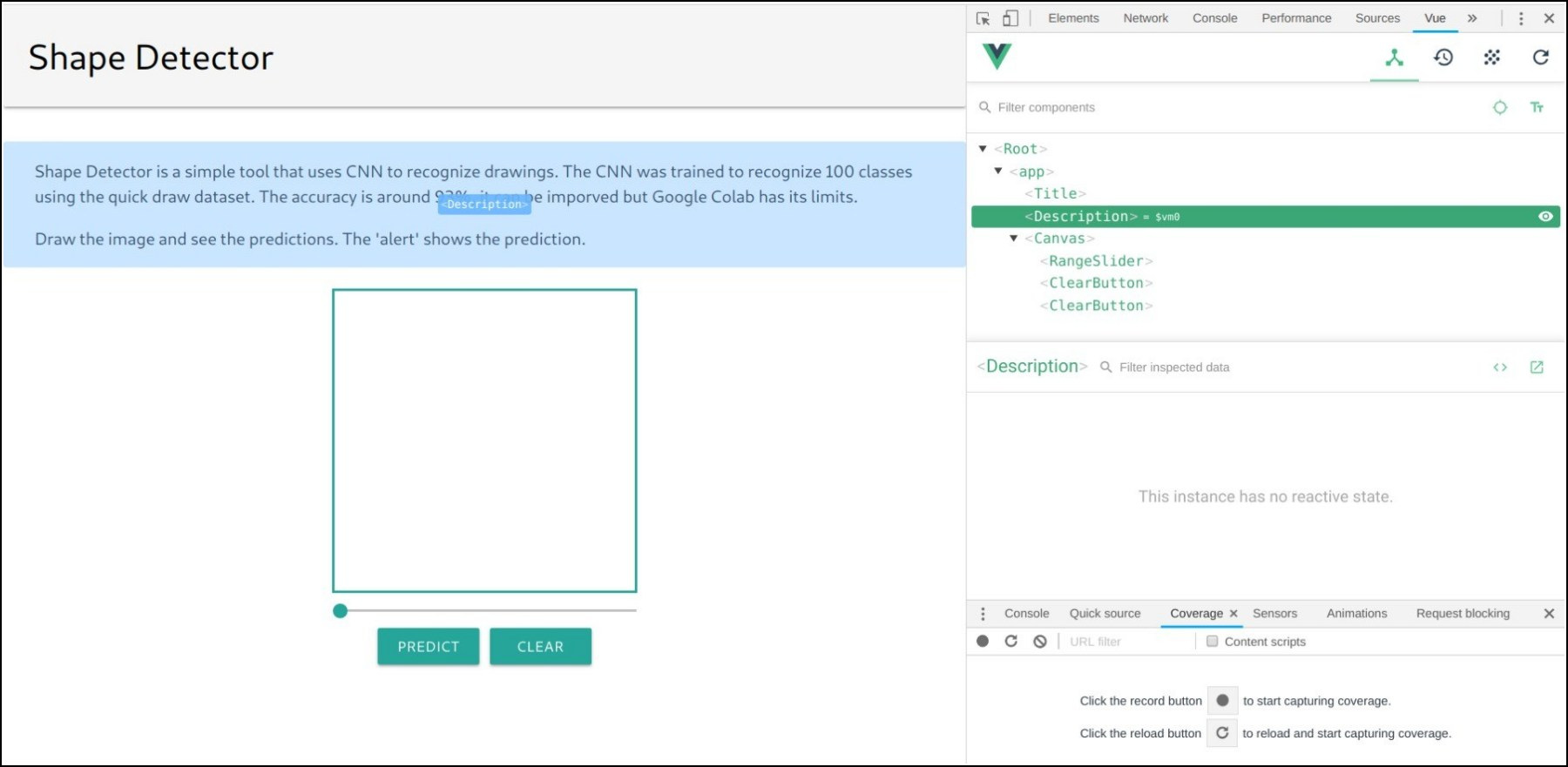‘ClearButton’ in the image should have been ‘CustomButton’.

• ‘Title’ and ‘Description’ are simple components and do not need explanation.
• The CustomButton component emits a `ButtonClick` event when clicked. Text in the button can be set in the parent component using a buttonText prop.
• The RangeSlider component emits an event `sliderInput` with slider’s current value as the event’s argument.

``````import * as tf from '@tensorflow/tfjs'

Vue.config.productionTip = false

new Vue({
render: h => h(App),
mounted: async function() {
Vue.prototype.\$model = model

let res = await fetch('model/class_names.txt') // load class_name file
let text = await res.text()
Vue.prototype.\$classArray = text.split('\n')
``````

Model weights and class_name file(file which tells class name corresponding to index) are loaded when the Root vue component is mounted. `Vue.prototype.\$model` and `Vue.prototype.\$classArray` are set so that they can be directly use in other components with `this.\$model` and `this.\$classArray`.

Loading model weights when ‘Root’ vue component in mounted, results in slow loading of the web page and long wait before First Meaningful Paint. This can be avoided by loading them after all components have been rendered, but I am too lazy to change the code once it has been written.

#### The Canvas component

This is the component where most of the frontend logic lies. The canvas size is set to `300x300` pixels.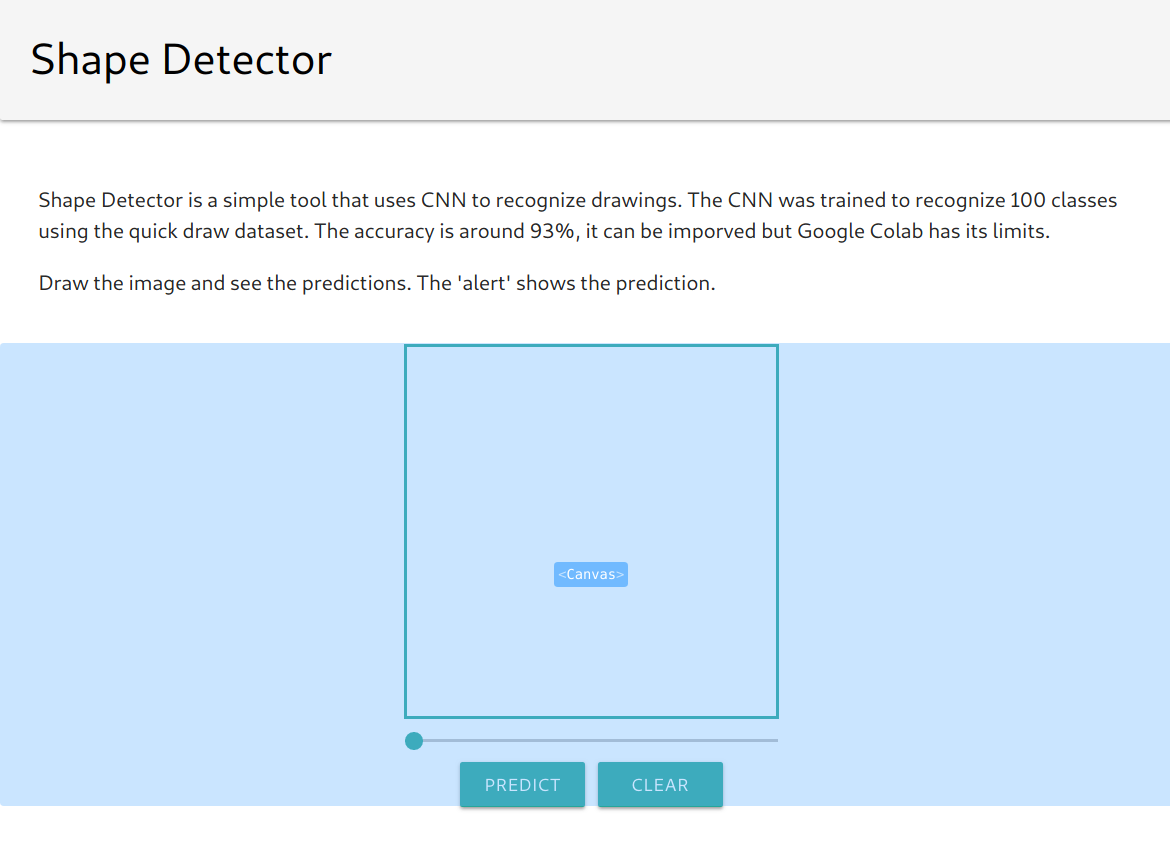``````data: function() {
return {
fabricCanvas: null,
canvasContext: null,
sliderStyle: {
width: '300px',
margin: 'auto',
}
}
}
``````

The `data` object in Canvas component has following properties:

• fabricCanvas – Current fabric canvas object
• canvasContext – The `canvas` element’s 2D rendering context
• sliderStyle – It is `RangeSlider` prop object bound to its `style` attribite

Then we have `setSliderValue`, `clearCanvas` and `maxIndex` methods to set canvas’ brush size, to clear the canvas and return index with maximum probability in an array respectively.

– Set background of canvas as white(`#ffffff`) after every clear operation else it will give wrong prediction everytime, as canvas’ default background is transparent.
`fabricCanvas.backgroundColor = '#ffffff'`

``````preprocessImage: function(imgData) {
return tf.tidy(()=>{
let tensor = tf.fromPixels(imgData, 1) // convert the image data to a tensor

// resize to 28 x 28
const resized = tf.image.resizeBilinear(tensor, [28, 28]).toFloat()

// Normalize the image
const offset = tf.scalar(255.0)
const normalized = tf.scalar(1.0).sub(resized.div(offset))

// insert a dimension of 1 into a tensor's shape
const batched = normalized.expandDims(0)
return batched
})
}
``````

In `preprocessImage` function, we take the current image from the canvas, convert it to a tensor, resize and normalize it. Finally, we add a dimension of 1 to get the batch shape.

``````predictImage: function() {
// get the image from canvas
let image = this.canvasContext.getImageData(0, 0, this.fabricCanvas.getWidth(), this.fabricCanvas.getHeight())

// preprocess the image and prediction
let pred = this.\$model.predict(this.preprocessImage(image)).dataSync()

// get array index with max probability
let maxIndex = this.maxIndex(pred)
}
``````

`model.predict` returns the probabilities of each class. The prediction array(`pred`) has 100 elements. We show `classArray[maxIndex]` as the prediction to shape drawn in the canvas.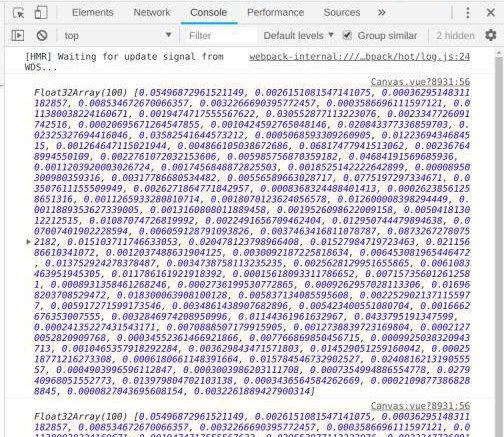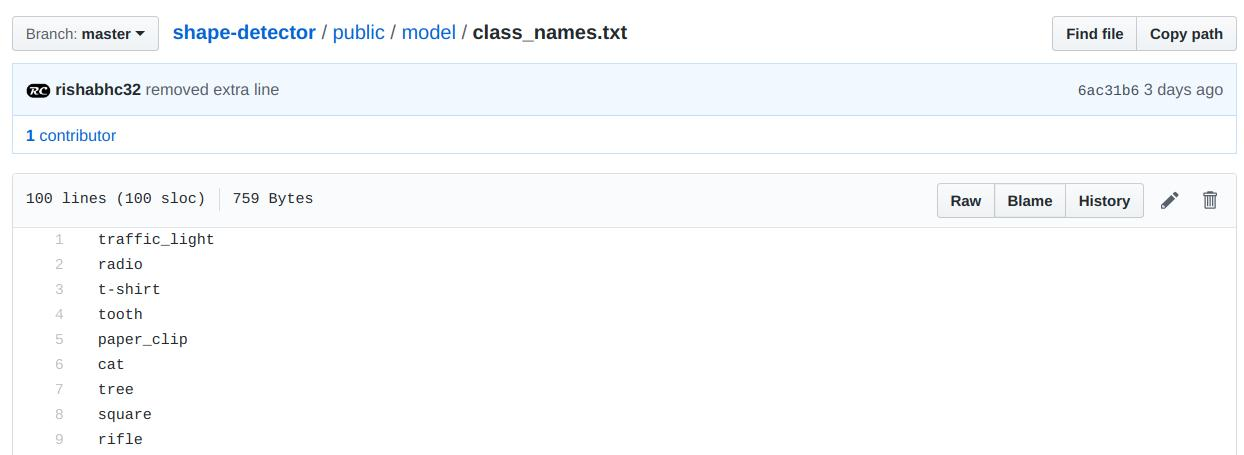## Testing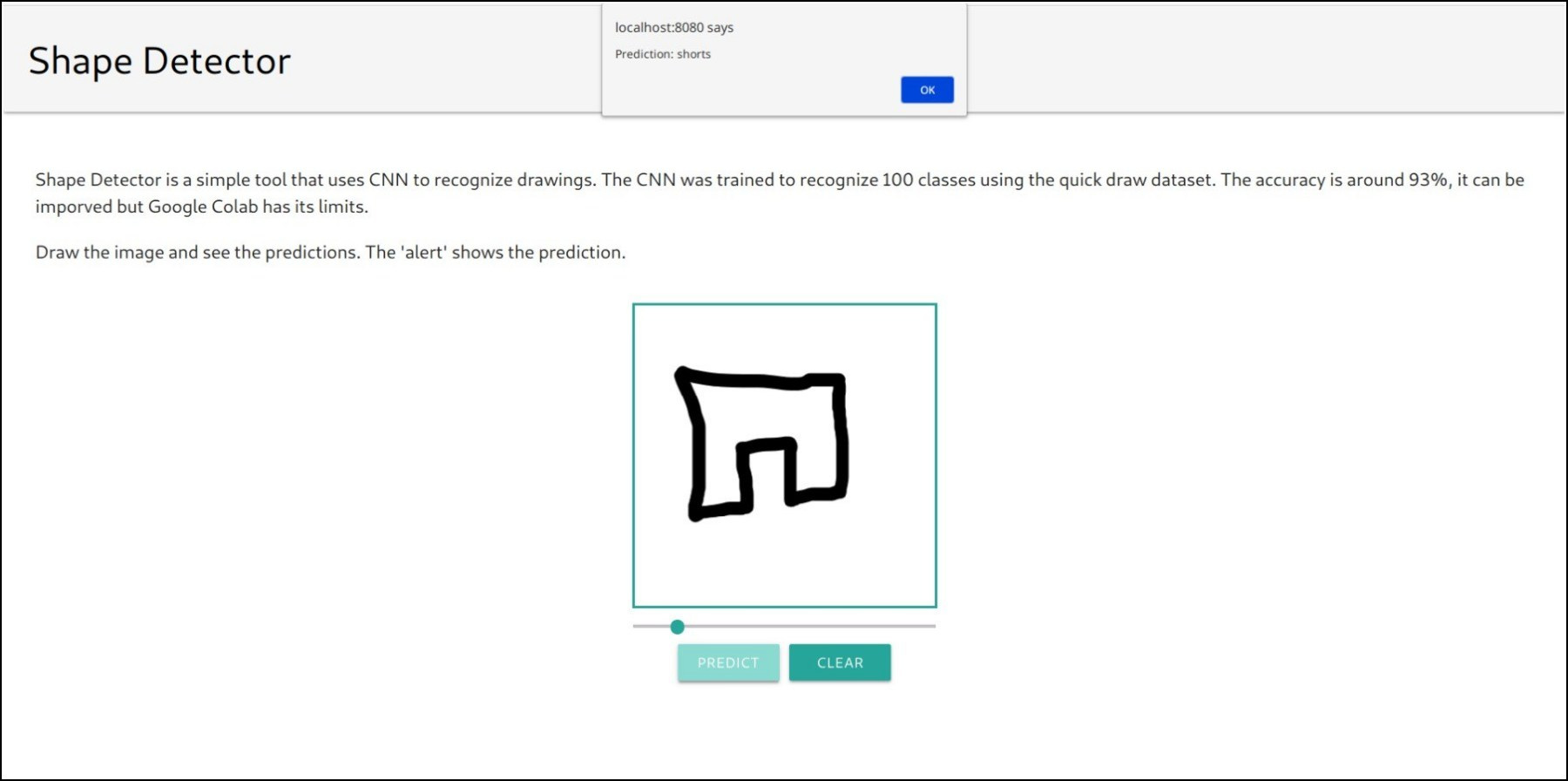Prediction: shorts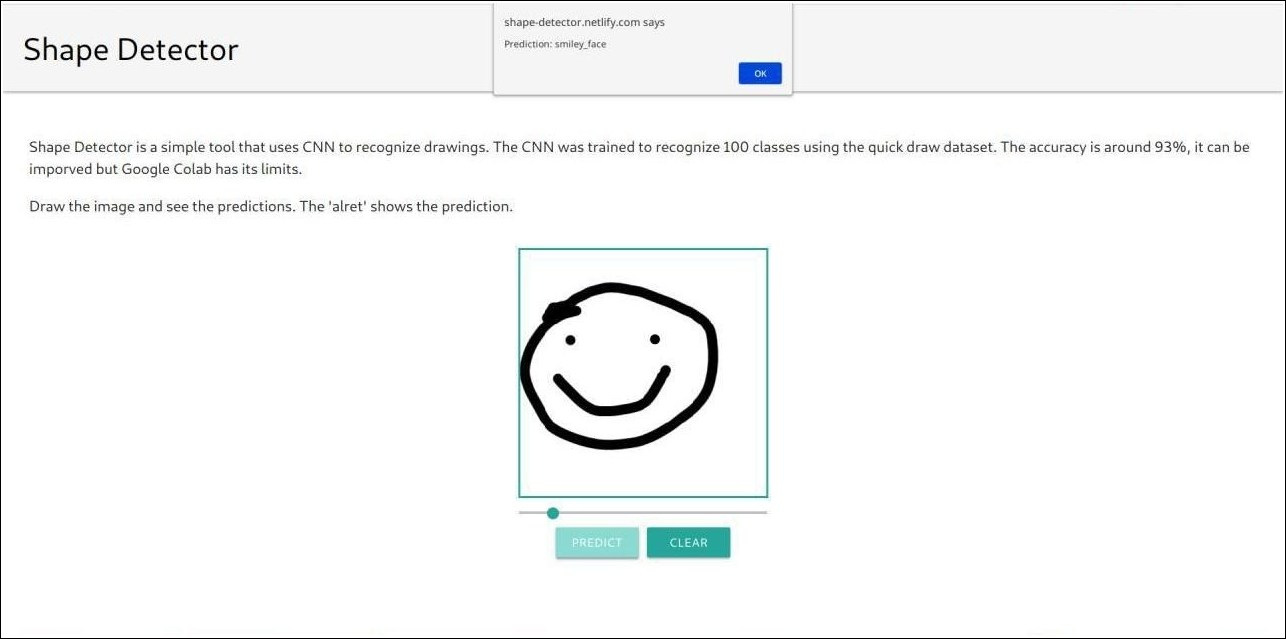Prediction: smiley_face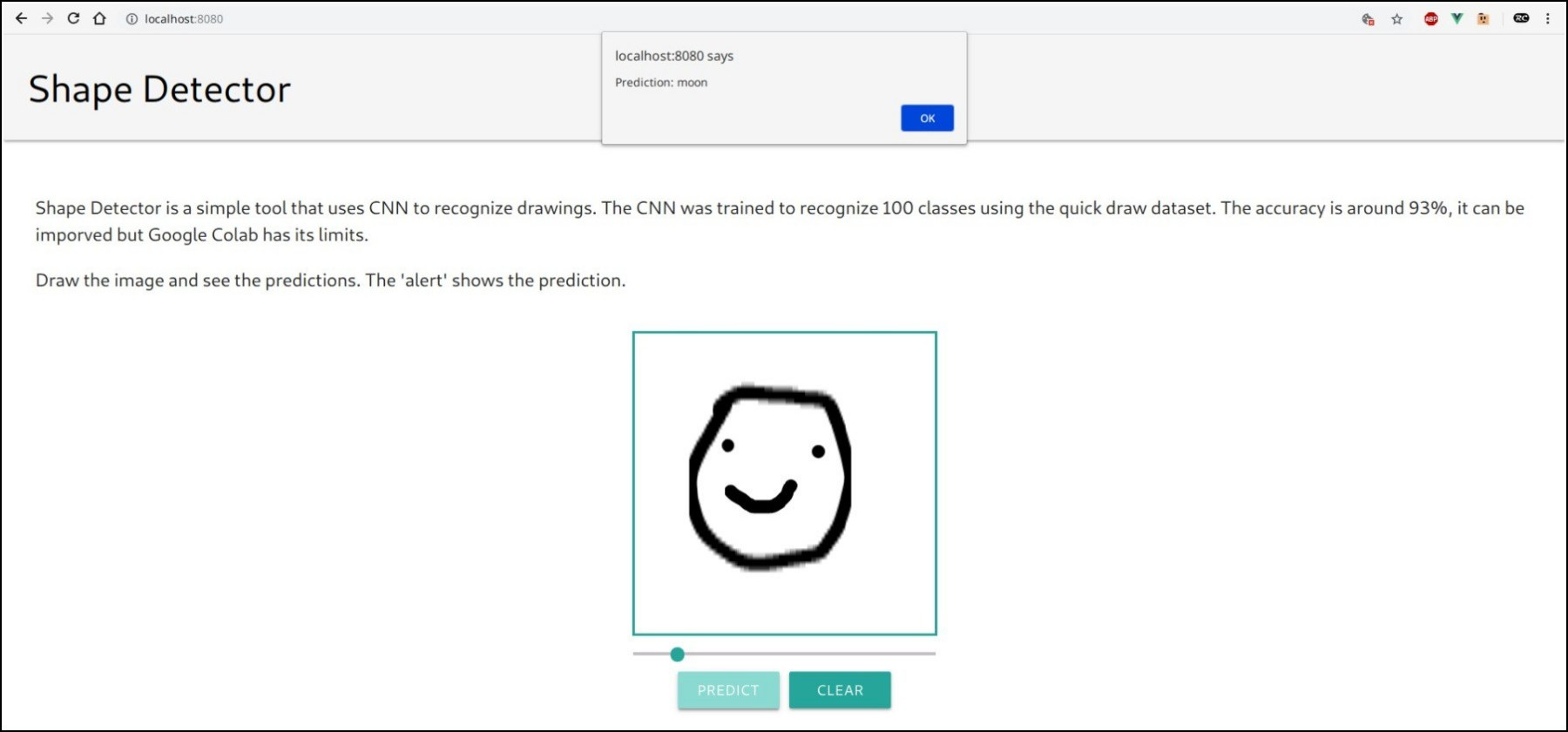Prediction: moon
It is not always accurate.

That’s all Fellas.

The code was written in 2 days after 15 days of controlled craving to write some code, so there might be slight hiccups in the program.